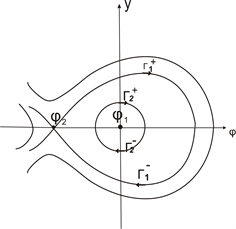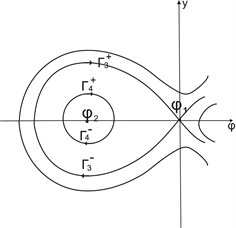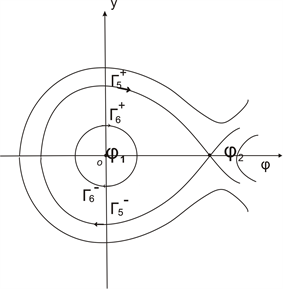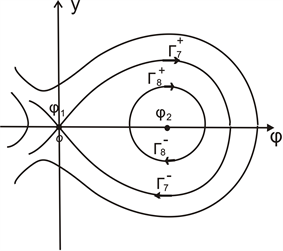﻿ (1 + 1)维Benjiamin Omo方程的精确行波解研究

# (1 + 1)维Benjiamin Omo方程的精确行波解研究Study of the Exact Traveling Wave Solutions of (1+1) Dimensional Benjiamin Omo Equation

Abstract: Through the traveling wave transformation, by exploiting the qualitative theory of differential equations and the bifurcation method of dynamical systems, we obtained some nonlinearwave solutions for Benjiamin Omo equation under different parameter forms after travelingwave transformation.

1. 研究背景和预备知识

${u}_{tt}+\beta {\left({u}^{2}\right)}_{xx}+\gamma {u}_{{}_{xxxx}}=0.$ (1)

(1 + 1) 维Benjiamin Omo方程    是物理学中一个十分重要的非线性发展方程，已经有许多人对这一模型进行了广泛的研究.如文献  中用基于改进的投影Riccati法求得了该模型的单变量行波解。文献  通过Jacobi椭圆函数展开法，求得了该方程的精确周期解。在文献   中，利用双线性方法和扩展的测试函数求得了其周期孤波解和双周期孤波解。文献  中通过改进的辅助方程法，求得了该方程的精确解。而本文运用平面动力系统的分支方法定性理论 ，讨论方程(1)的非线性波解。

${c}^{2}{\phi }^{″}+2\beta {\left({\phi }^{\prime }\right)}^{2}+2\beta \phi {\phi }^{″}+\gamma {\phi }^{‴}\text{'}=0.$ (2)

${c}^{2}\phi +\beta {\phi }^{2}+\gamma {\phi }^{″}=0.$ (3)

${\phi }^{\prime }=y$，得到下面的平面系统

$\left\{\begin{array}{l}\frac{\text{d}\phi }{\text{d}\xi }=y\\ \frac{\text{d}y}{\text{d}\xi }=-\frac{1}{\gamma }\left(\beta {\phi }^{2}+{c}^{2}\phi \right).\end{array}$ (4)

$H\left(\phi ,y\right)={y}^{2}+\frac{2\beta }{3\gamma }{\phi }^{3}+\frac{{c}^{2}}{\gamma }{\phi }^{2}.$ (5)

$\Delta =\frac{{c}^{4}}{{\gamma }^{2}}$

$\Delta >0$ 时， $f\left(\phi \right)$ 有两个零点 ${\phi }_{1}$${\phi }_{2}$，它们的表达式为

${\phi }_{1}=0,{\phi }_{2}=\frac{-{c}^{2}}{\beta }.$ (6)

$\Delta \le 0$ 时， $f\left(\phi \right)$ 没有零点。

$\lambda =±\sqrt{{f}^{\prime }\left(\stackrel{˜}{\phi }\right)}.$

(1) 如果 ${f}^{\prime }\left(\stackrel{˜}{\phi }\right)>0$，则 $\left(\stackrel{˜}{\phi },0\right)$ 是一个鞍点。

(2) 如果 ${f}^{\prime }\left(\stackrel{˜}{\phi }\right)<0$，则 $\left(\stackrel{˜}{\phi },0\right)$ 是一个中心。

(3) 如果 ${f}^{\prime }\left(\stackrel{˜}{\phi }\right)=0$，则 $\left(\stackrel{˜}{\phi },0\right)$ 是一个退化鞍点。

2. Benjiamin Omo方程的行波解的参数表达式

${u}_{1}^{±}=p\left(1-3{\mathrm{tanh}}^{2}\left(\frac{1}{2}{\eta }_{1}|\xi |\right)\right)$ (7)

${u}_{2}^{±}=\frac{{a}_{2}\left({a}_{3}-{a}_{1}\right)-{a}_{2}\left({a}_{3}-{a}_{2}\right)s{n}^{2}\left(\sqrt{\frac{\beta \left({a}_{3}-{a}_{1}\right)}{6\gamma }}|\xi |,\sqrt{\frac{{a}_{3}-{a}_{2}}{{a}_{3}-{a}_{1}}}\right)}{{a}_{3}-{a}_{1}-\left({a}_{3}-{a}_{2}\right)s{n}^{2}\left(\sqrt{\frac{\beta \left({a}_{3}-{a}_{1}\right)}{6\gamma }}|\xi |,\sqrt{\frac{{a}_{3}-{a}_{2}}{{a}_{3}-{a}_{1}}}\right)}$ (8)

$p=\frac{{c}^{2}}{2\beta },$

${\eta }_{1}=\sqrt{\frac{{c}^{2}}{\gamma }},$

${a}_{1}+{a}_{2}+{a}_{3}=-\frac{3{c}^{2}}{2\beta },$

${a}_{1}{a}_{2}+{a}_{1}{a}_{3}+{a}_{2}{a}_{3}=0,$

${a}_{1}{a}_{2}{a}_{3}=0.$

${\Gamma }_{1}^{±}:{y}^{2}=\frac{2\beta }{3\gamma }\left(-{\phi }^{3}-\frac{3{c}^{2}}{2\beta }{\phi }^{2}+\frac{3\gamma }{2\beta }{h}_{1}\right)=\frac{2\beta }{3\gamma }{\left(\phi -{\phi }_{2}\right)}^{2}\left(p-\phi \right)$ (9)

$\frac{\text{d}\phi }{\text{d}\xi }=±\sqrt{\frac{2\beta }{3\gamma }{\left(\phi -{\phi }_{2}\right)}^{2}\left(p-\phi \right)}$ (10)Figure 1. Track chart of the ${\Gamma }_{1}^{±}$ and ${\Gamma }_{2}^{±}$

${\int }_{{\phi }_{2}}^{\phi }\frac{\text{d}\phi }{\left(\phi -{\phi }_{2}\right)\sqrt{p-\phi }}=±{\int }_{0}^{\xi }\sqrt{\frac{2\beta }{3\gamma }}\text{d}\xi$ (11)

${\Gamma }_{2}^{±}:{y}^{2}=\frac{2\beta }{3\gamma }\left(-{\phi }^{3}-\frac{3{c}^{2}}{2\beta }{\phi }^{2}\right)=\frac{2\beta }{3\gamma }\left(\phi -{a}_{1}\right)\left(\phi -{a}_{2}\right)\left({a}_{3}-\phi \right)$ (12)

$\frac{\text{d}\phi }{\text{d}\xi }=±\sqrt{\frac{2\beta }{3\gamma }\left(\phi -{a}_{1}\right)\left(\phi -{a}_{2}\right)\left({a}_{3}-\phi \right)}.$ (13)

${\int }_{{a}_{2}}^{\phi }\frac{\text{d}\phi }{\sqrt{\left(\phi -{a}_{1}\right)\left(\phi -{a}_{2}\right)\left({a}_{3}-\phi \right)}}=±{\int }_{0}^{\xi }\sqrt{\frac{2\beta }{3\gamma }}\text{d}\xi .$ (14)

${u}_{3}^{±}=\frac{3{c}^{2}}{2\beta }\left({\mathrm{tanh}}^{2}\left(\frac{1}{2}{\eta }_{2}|\xi |\right)-1\right)$ (15)

${u}_{4}^{±}=\left({a}_{2}-{a}_{1}\right)s{n}^{2}\left(\sqrt{\frac{-\beta \left({a}_{3}-{a}_{1}\right)}{6\gamma }}|\xi |,\sqrt{\frac{{a}_{2}-{a}_{1}}{{a}_{3}-{a}_{1}}}\right)+{a}_{1}$ (16)

${\Gamma }_{3}{}^{±}:{y}^{2}=\frac{-2\beta }{3\gamma }\left({\phi }^{3}+\frac{3{c}^{2}}{2\beta }{\phi }^{2}\right)=-\frac{2\beta }{3\gamma }{\phi }^{2}\left(\phi +\frac{3{c}^{2}}{2\beta }\right)$ (17)Figure 2. Track chart of the ${\Gamma }_{3}^{±}$ and ${\Gamma }_{4}^{±}$

$\frac{\text{d}\phi }{\text{d}\xi }=±\sqrt{-\frac{2\beta }{3\gamma }{\phi }^{2}\left(\phi +\frac{3{c}^{2}}{2\beta }\right)}$ (18)

${\int }_{\frac{-3{c}^{2}}{2\beta }}^{\phi }\frac{\text{d}\phi }{-\phi \sqrt{\phi +\frac{3{c}^{2}}{2\beta }}}=±{\int }_{0}^{\xi }\sqrt{-\frac{2\beta }{3\gamma }}\text{d}\xi$ (19)

${\Gamma }_{4}^{±}:{y}^{2}=-\frac{2\beta }{3\gamma }\left({\phi }^{3}+\frac{3{c}^{2}}{2\beta }{\phi }^{2}-\frac{3\gamma }{2\beta }{h}_{1}\right)=\frac{-2\beta }{3\gamma }\left(\phi -{a}_{1}\right)\left({a}_{2}-\phi \right)\left({a}_{3}-\phi \right)$ (20)

$\frac{\text{d}\phi }{\text{d}\xi }=±\sqrt{-\frac{2\beta }{3\gamma }\left(\phi -{a}_{1}\right)\left({a}_{2}-\phi \right)\left({a}_{3}-\phi \right)}$ (21)

${\int }_{{a}_{1}}^{\phi }\frac{\text{d}\phi }{\sqrt{\left(\phi -{a}_{1}\right)\left({a}_{2}-\phi \right)\left({a}_{3}-\phi \right)}}=±{\int }_{0}^{\xi }\sqrt{\frac{-2\beta }{3\gamma }}\text{d}\xi$ (22)

${\Gamma }_{5}^{±}:{y}^{2}=\frac{-2\beta }{3\gamma }\left({\phi }^{3}+\frac{3{c}^{2}}{2\beta }{\phi }^{2}-\frac{3\gamma }{2\beta }{h}_{1}\right)=-\frac{2\beta }{3\gamma }{\left({\phi }_{2}-\phi \right)}^{2}\left(\phi -p\right)$ (23)Figure 3. Track chart of the ${\Gamma }_{5}^{±}$ and ${\Gamma }_{6}^{±}$

$\frac{\text{d}\phi }{\text{d}\xi }=±\sqrt{-\frac{2\beta }{3\gamma }{\left({\phi }_{2}-\phi \right)}^{2}\left(\phi -p\right)}$ (24)

${\Gamma }_{6}^{±}:{y}^{2}=-\frac{2\beta }{3\gamma }\left({\phi }^{3}+\frac{3{c}^{2}}{2\beta }{\phi }^{2}\right)=\frac{-2\beta }{3\gamma }\left(\phi -{a}_{1}\right)\left({a}_{2}-\phi \right)\left({a}_{3}-\phi \right)$ (25)

$\frac{\text{d}\phi }{\text{d}\xi }=±\sqrt{-\frac{2\beta }{3\gamma }\left(\phi -{a}_{1}\right)\left({a}_{2}-\phi \right)\left({a}_{3}-\phi \right)}$ (26)

${\Gamma }_{7}^{±}:{y}^{2}=\frac{2\beta }{3\gamma }\left(-{\phi }^{3}-\frac{3{c}^{2}}{2\beta }{\phi }^{2}\right)=\frac{2\beta }{3\gamma }{\phi }^{2}\left(-\frac{3{c}^{2}}{2\beta }-\phi \right)$ (27)

$\frac{\text{d}\phi }{\text{d}\xi }=±\sqrt{\frac{2\beta }{3\gamma }{\phi }^{2}\left(-\frac{3{c}^{2}}{2\beta }-\phi \right)}$ (28)Figure 4. Track chart of the ${\Gamma }_{7}^{±}$ and ${\Gamma }_{8}^{±}$

${\Gamma }_{8}^{±}:{y}^{2}=\frac{2\beta }{3\gamma }\left(-{\phi }^{3}-\frac{3{c}^{2}}{2\beta }{\phi }^{2}+\frac{3\gamma }{2\beta }{h}_{1}\right)=\frac{2\beta }{3\gamma }\left(\phi -{a}_{1}\right)\left(\phi -{a}_{2}\right)\left({a}_{3}-\phi \right)$ (29)

$\frac{\text{d}\phi }{\text{d}\xi }=±\sqrt{\frac{2\beta }{3\gamma }\left(\phi -{a}_{1}\right)\left(\phi -{a}_{2}\right)\left({a}_{3}-\phi \right)}.$ (30)

NOTES

*通讯作者。

 Benjiamin, T.B. (1967) Internal Waves of Permanent Formin Fluids of Great Depth. Journal of Fluid Mechanics, 29, 559-592.
https://doi.org/10.1017/S002211206700103X

 Davies, R.E. and Acrivos, A. (1967) Solitary Internal Waves Indeep Water. Journal of Fluid Mechanics, 29, 593-607.
https://doi.org/10.1017/S0022112067001041

 Ono, H. (1975) Algebraic Solitary Waves in Stratifiedfluids. Journal of the Physical Society of Japan, 39, 1082-1091.
https://doi.org/10.1143/JPSJ.39.1082

 Wang, Z., Li, D.-S., Lu H.-F., et al. (2005). A Method for Constructing Exact Solutions and Application to Benjamin Ono Equation. Chinese Physics, 14, 2158-2158.
https://doi.org/10.1088/1009-1963/14/11/003

 Fu, Z. and Liu, S. (2003) The JEFE Method and Periodic Solutions of Two Kinds of Nonlinear Wave Equations. Communications in Nonlinear Science and Numerical Simulation, 8, 67-75.
https://doi.org/10.1016/S1007-5704(02)00082-5

 Xu, Z.H., Xian, D.Q. and Chen, H.L. (2010) New Periodic Solitary-Wave Solutions for the Benjiamin Ono Equation. Applied Mathematics and Computation, 215, 4439-4442.
https://doi.org/10.1016/j.amc.2009.11.009

 许瑞麟, 许晓革, 孟祥花. (1+1)维BenjiaminOno方程的精确显式解[J]. 北京信息科技大学学报(自然科学版), 2011, 26(3): 58-61.

 杨娟, 冯庆江, 曾春花. 一类非线性数学物理方程的精确解[J]. 数学的实践与认识, 2018, 48(17): 309-313.

 Wu, Y., Liu, Z.R. and Bai, C.Z. (2013) New Types of Nonlinear Waves and Bifurcation Phenomena in Schamel-Korteweg-de Vries Equation. Abstract and Applied Analysis, 2013, 1056-1083.
https://doi.org/10.1155/2013/483492

Top